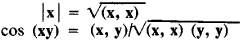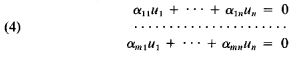vector space

(redirected from Abstract vector space)
Also found in: Dictionary.

vector space

[′vek·tər ‚spās]
(mathematics)
A system of mathematical objects which have an additive operation producing a group structure and which can be multiplied by elements from a field in a manner similar to contraction or magnification of directed line segments in euclidean space. Also known as linear space.

Vector Space

a mathematical concept that generalizes the concept of a set of all (free) vectors of ordinary three-dimensional space.

Definition The rules for adding vectors and multiplying them by real numbers are specified for vectors of three-dimensional space. As applied to any vectors x, y, and z and any numbers α and β , these rules satisfy the following conditions (conditions A):

(1) x + y = y + x (commutation in addition);

(2) (x + y) + z = x + (y + z) (association in addition);

(3) there is a zero vector, 0, which satisfies the condition x + 0 = x for any vector x;

(4) for any vector x there is a vector y inverse to it such that x + y = 0;

(5) 1 · x = x;

(6) α(βx) = (±²) x (association in multiplication);

(7) (α + β)x = αx + βx (distributive property with respect to a numerical multiplier); and

(8) α(x + y) = αβ + αy (distributive property with respect to a vector multiplier).

A vector (or linear) space is a set R consisting of elements of any type (called vectors) in which the operations of addition and multiplication of elements by real numbers satisfy conditions A (conditions (l)-(4) express the fact that the operation of addition defined in a vector space transforms it into a commutative group).

The expression

(1) α1e1 + α2e2 + … αnen

is called a linear combination of the vectors e1, e2, … en with coefficients α1, α2, … αn Linear combination (1) is called nontrivial if at least one of the coefficients α2, … αn differs from zero. The vectors e1, e2, … en are called linearly dependent if there exists a nontrivial combination (1) representing a zero vector. In the opposite case—that is, if only a trivial combination of vectors e1, e2, … en is equal to the zero vector— the vectors e1, e2, … en are called linearly independent.

The vectors (free) of three-dimensional space satisfy the following condition (condition B): there exist three linearly independent vectors and any four vectors are linearly dependent (any three nonzero vectors that do not lie in the same plane are linearly independent).

A vector space is called n-dimensional (or has “dimension n ”) if there are n linearly independent elements e1, e2, … en in it and if any n + 1 elements are linearly dependent (generalized condition B). A vector space is called infinite dimensional if for any natural number n in it there are n linearly independent vectors. Any n linearly independent vectors of an n-dimensional vector space form the basis of this space. If e1, e2, … en form the basis of a vector space, then any vector x of this space can be represented uniquely in the form of a linear combination of basis vectors:

x = α1e1, α2e2, … αnxen

Here, the numbers α1, α2, … αn are called coordinates of vector x in the given basis.

Examples The set of all vectors of three-dimensional space obviously forms a vector space. A more complex example is the so-called n-dimensional arithmetic space. The vectors of this space are ordered systems of n real numbers: (λ1, λ2, …, λn). The sum of two vectors and the product of a vector and a number are defined by the relations

1, λ2, … λn) + (μ1, μ2, … μn) = (λ1 + μ12 + μ2, …, λn + μn)

λ(λ1, λ2, …, λn) = (αλ1, αλ2,… αλn)

As the basis of this space, one can use, for example, the following system of n vectors: e1 = (1, 0, … , 0), e2 = (0, 1, … , 0),… , en = (0, 0, … , 1).

The set R of all polynomials α0+ α1γ + … + αnγn (of any degree n) of a single variable with real coefficients α0, α1, … αn and with the ordinary algebraic rules for adding polynomials and multiplying them by real numbers forms a vector space. The polynomials 1, γ, γ2, … , γn (for any n) are linearly independent in R, and therefore R is an infinite-dimensional vector space. Polynomials of degree not higher than n form a vector space of dimension n + 1 ; the polynomials 1, γ, γ2, … , γn can serve as its basis.

Subspaces The vector space R’ is called a subspace of R if (1)x(that is, every vector of space R′ is also a vector of space R) and (2) for every vector ∊ and for every two vectors v1 and v2 ∊, vector セv (for any セ) and vector v1 + v2 are one and the same, regardless of whether one considers vectors v, v1, and v2 as elements of space R′ or R . The linear manifold of vectors xlx2, … , xp is the set of all the possible linear combinations of these vectors, that is, of vectors of the form α1x1 + α2x2 · · · αpxp . In three-dimensional space, the linear manifold of a single, nonzero vector x1 will obviously be the set of all vectors lying on the straight line determined by the vector x1. The linear manifold of two vectors x1 and x2, not lying on the same straight line, will be the set of all vectors located in the plane determined by vectors x1 and x2. In the general case of an arbitrary vector space R, the linear manifold of vectors x1, x2, …, xp of this space is a subspace of space R of dimension K≥ p. In an n-dimensional vector space there are subspaces of all dimensions less than n. Each finite-dimensional (of given dimension k) subspace R′ of vector space R is the linear manifold of any k linearly independent vectors lying within R′. A space consisting of all polynomials of degree ≥ n (the linear manifold of the polynomials 1, γ, γ2, … , γn) is an (n + 1)-dimensional subspace of space R of all polynomials.

Euclidean spaces In order to develop geometric methods in the theory of vector spaces, it has been necessary to find methods of generalizing such concepts as the length of a vector and the angle between vectors. One of the possible methods consists in the fact that any two vectors x and y of R are set in correspondence with a number designated as (x,y) and called the scalar product of vectors x and y. It is necessary to satisfy the following axioms for the scalar product:

(1) (x, y) = (y, x) (commutativity);

(2) (x1 + x2, y) = (x1, y) + (x2, y) (distributive property);

(3) (αx, y) = α(x, y); and

(4) (x, x) 0 for any x, while (x, x) = 0 only for x = 0.

The ordinary scalar product in three-dimensional space satisfies these axioms. A vector space, in which a scalar product satisfying the above axioms is defined is called a Euclidean space; it can be either finite in dimensions (n -dimensional) and infinite in dimensions. An infinite-dimensional Euclidean space is usually called a Hilbert space. The length |x | of vector x and the angle (xy) between vectors x and y of a Euclidean space are defined by the scalar product according to the formulasAn example of a Euclidean space is an ordinary three-dimensional space with the scalar product defined in vector calculus. Euclidean n-dimensional (arithmetic) space En is obtained by defining, in an n-dimensional arithmetic vector space, the scalar product of vectors x = (λ1, … ,λn) and y = (μ1, … , μn) by the relation

(2) (x, y) = λ1μ1 + λ2μ2 + … + λnμn

Requirements (l)-(4) are clearly fulfilled here.

In Euclidean spaces the concept of orthogonal (perpendicular) vectors is introduced. Precisely, vectors x and y are called orthogonal if their scalar product is equal to zero: (x,y) = 0. In the space En considered here, the condition of orthogonality of vectors x = (λ,… , λn) and y = (μ1, …, μn, as follows from relation (2), has the form

(3) λ1μ1 + λ2μ2 + … + λnμn = 0

Applications The concept of a vector space (and various generalizations) is widely used in mathematics and has applications in the natural sciences. For example, let R be the set of all the solutions of the linear homogeneous differential equation yn +a1(x)yn-1 + … + an (x)y = 0. Clearly, the sum of two solutions and the product of a solution times a number are also solutions to this equation. Thus, R satisfies conditions A. It has been proved that for R the generalized condition B is fulfilled. Consequently, R is a vector space. Any basis in the vector space under consideration is called a fundamental system of solutions, knowledge of which permits one to find all the solutions to the equation under consideration. The concept of a Euclidean space permits the complete geometrization of the theory of systems of homogeneous linear equations:Let us consider, in the Euclidean space E′ , vectors ai = (αi 1, α2 2, … αi n), where i = 1,2, … , n , and the solution vector μ = (μl μ2, … , μn). Using equation (2) for the scalar product of the vectors of En we give equations (4) the form

(5) (ai , u) = 0, i = 1, 2, …, m

From relations (5) and equation (3), it follows that the solution vector u is orthogonal to all vectors ai . In other words, this vector is orthogonal to the linear manifold of vectors ai ; that is, the solution u is any vector of the orthogonal complement of the linear manifold of vectors ai . Infinite-dimensional linear spaces play an important role in mathematics and physics. An example of such a space is the space C of continuous functions on a segment with the usual operations of addition and multiplication on real numbers. The space of all polynomials mentioned previously is a sub-space of space C .

REFERENCES

Aleksandrov, P. S. Lektsii po analiticheskoi geometrii. Moscow, 1968.
Gel’fand, I. M. Lektsii po lineinoi algebre. Moscow-Leningrad, 1948.

E. G. POZNIAK

vector space

(mathematics)
An additive group on which some (scalar) field has an associative multiplicative action which distributes over the addition of the vector space and respects the addition of the (scalar) field: for vectors u, v and scalars h, k; h(u+v) = hu + hv; (h+k)u = hu + ku; (hk)u = h(ku).

Site: Follow: Share:
Open / Close